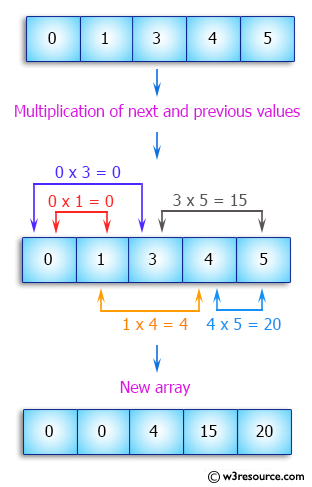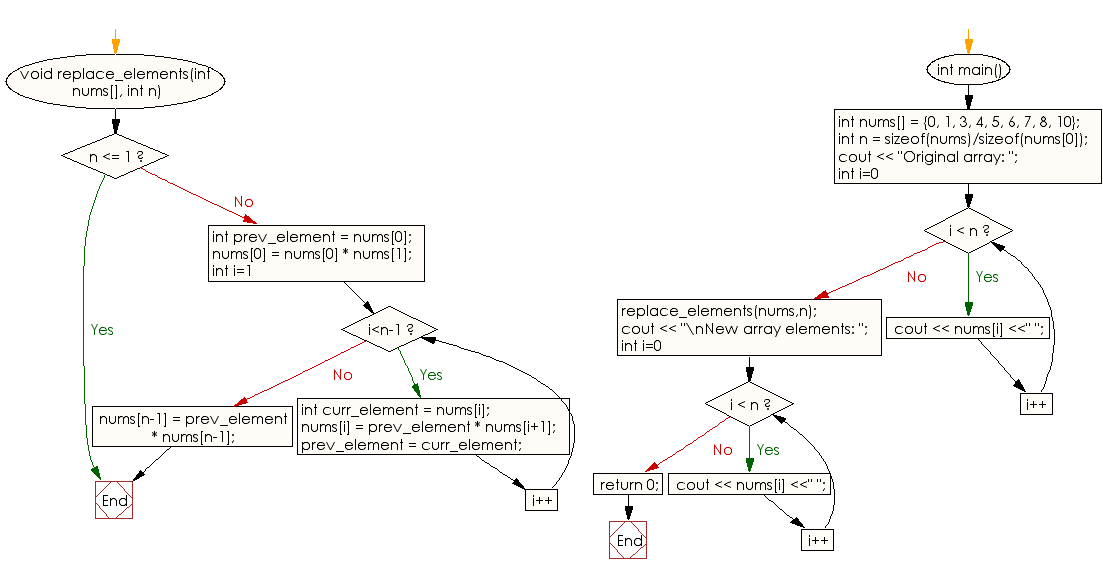﻿ C++ : Multiply next, previous values to update array values# C++ Exercises: Update every array element by multiplication of next and previous values of a given array of integers

## C++ Array: Exercise-11 with Solution

Write a C++ program to update every array element by multiplication of next and previous values of a given array of integers.

Pictorial Presentation:Sample Solution:

C++ Code :

``````#include<iostream>
using namespace std;

void replace_elements(int nums[], int n)
{
if (n <= 1)
return;

int prev_element = nums;
nums = nums * nums;

for (int i=1; i<n-1; i++)
{
int curr_element = nums[i];

nums[i] = prev_element * nums[i+1];

prev_element = curr_element;
}

nums[n-1] = prev_element * nums[n-1];
}

int main()
{
int nums[] = {0, 1, 3, 4, 5, 6, 7, 8, 10};
int n = sizeof(nums)/sizeof(nums);
cout << "Original array: ";
for (int i=0; i < n; i++)
cout << nums[i] <<" ";
replace_elements(nums,n);
cout << "\nNew array elements: ";
for (int i=0; i < n; i++)
cout << nums[i] <<" ";
return 0;

}
``````

Sample Output:

```Original array: 0 1 3 4 5 6 7 8 10
New array elements: 0 0 4 15 24 35 48 70 80
```

Flowchart:C++ Code Editor:

What is the difficulty level of this exercise?

Test your Programming skills with w3resource's quiz.

﻿